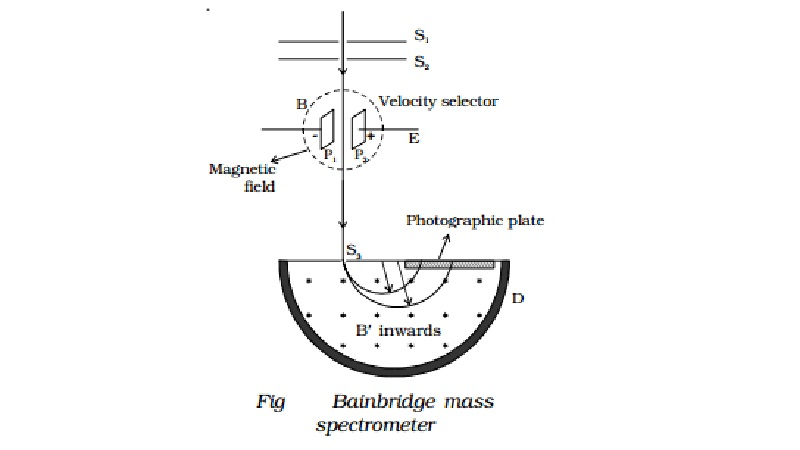Home | | Physics | | Physics | Bainbridge mass spectrometer - Determination of isotopic masses of nuclei

# Bainbridge mass spectrometer - Determination of isotopic masses of nucleiBainbridge mass spectro-meter is an instrument used for the accurate determination of atomic masses. A schematic diagram of this spectrometer is shown in Fig.

Bainbridge mass spectrometer - Determination of isotopic masses of nuclei

Bainbridge mass spectro-meter is an instrument used for the accurate determination of atomic masses. A schematic diagram of this spectrometer is shown in Fig.Atoms  with  one  or  more electrons  removed,  have  a  net positive charge and they become positive ions. A beam of positive ions produced in a discharge tube is collimated into a fine beam by two narrow      slits   S1  and       S2. This fine  beam        enters  into  a       velocity selector. The velocity selector allows the ions of a particular velocity to come out of it, by the combined action of an electric and a magnetic field. The velocity selector consists of two plane parallel plates P1 and P2, which produces a uniform electric field E and an electromagnet, to produce uniform magnetic field B (represented by the dotted circle). These two fields are at right angles to each other and to the direction of the beam.

The electric field and magnetic field are so adjusted that the deflection produced by one field is nullified by the other, so that the ions do not suffer any deflection within the velocity selector. Let E and B be the electric field intensity and magnetic induction respectively and q be the charge of the positive ion. The force exerted by the electric field is equal to qE and the force exerted by the magnetic field is equal to Bqv where v is the velocity of the positive ion.

qE = Bqv

v = E/B

Only those ions having this velocity v, pass out of the velocity selector and then through the slit S3, to enter the evacuated

chamber D. These positive ions having the same velocity are subjected to another strong uniform magnetic field of induction B at right angles to the plane of the paper acting inwards. These ions are deflected along circular path of radius R and strike the photographic plate. The force due to magnetic field Bqv provides the centripetal force.

Bqv = mv2

R

B qR m = v

Substituting v = E/B

m = BB qR

E

Ions with different masses trace semi-circular paths of different radii and produce dark lines on the plate. The distance between the opening of the chamber and the position of the dark line gives the diameter 2R from which radius R can be calculated.

Since q, B, B, E and R are known, the mass of the positive ions and hence isotopic masses can be calculated.

Study Material, Lecturing Notes, Assignment, Reference, Wiki description explanation, brief detail

Related Topics